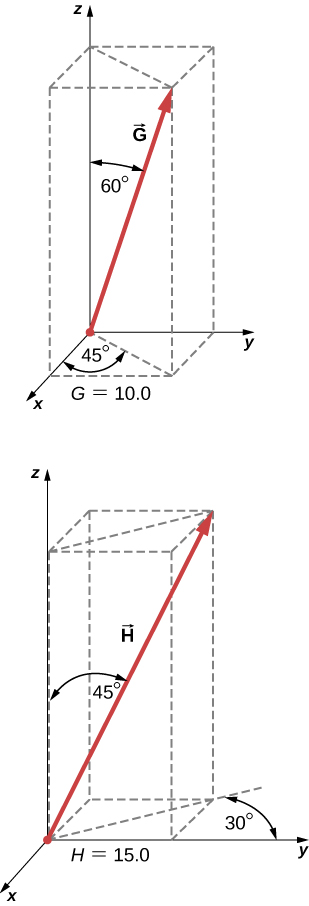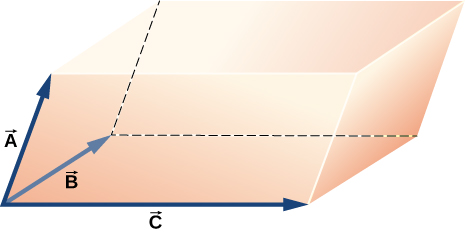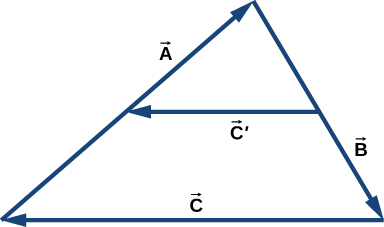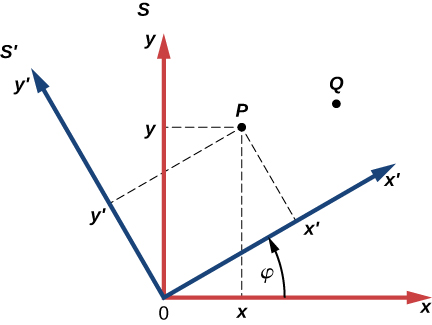# 2.4 Products of vectors  (Page 8/16)

 Page 10 / 16

A skater glides along a circular path of radius 5.00 m in clockwise direction. When he coasts around one-half of the circle, starting from the west point, find (a) the magnitude of his displacement vector and (b) how far he actually skated. (c) What is the magnitude of his displacement vector when he skates all the way around the circle and comes back to the west point?

a. 10.00 m, b. $5\pi \phantom{\rule{0.2em}{0ex}}\text{m}$ , c. 0

A stubborn dog is being walked on a leash by its owner. At one point, the dog encounters an interesting scent at some spot on the ground and wants to explore it in detail, but the owner gets impatient and pulls on the leash with force $\stackrel{\to }{F}=\left(98.0\stackrel{^}{i}+132.0\stackrel{^}{j}+32.0\stackrel{^}{k}\right)\text{N}$ along the leash. (a) What is the magnitude of the pulling force? (b) What angle does the leash make with the vertical?

If the velocity vector of a polar bear is $\stackrel{\to }{u}=\left(-18.0\stackrel{^}{i}-13.0\stackrel{^}{j}\right)\text{km}\text{/}\text{h}$ , how fast and in what geographic direction is it heading? Here, $\stackrel{^}{i}$ and $\stackrel{^}{j}$ are directions to geographic east and north, respectively.

22.2 km/h, $35.8\text{°}$ south of west

Find the scalar components of three-dimensional vectors $\stackrel{\to }{G}$ and $\stackrel{\to }{H}$ in the following figure and write the vectors in vector component form in terms of the unit vectors of the axes.A diver explores a shallow reef off the coast of Belize. She initially swims 90.0 m north, makes a turn to the east and continues for 200.0 m, then follows a big grouper for 80.0 m in the direction $30\text{°}$ north of east. In the meantime, a local current displaces her by 150.0 m south. Assuming the current is no longer present, in what direction and how far should she now swim to come back to the point where she started?

240.2 m, $2.2\text{°}$ south of west

A force vector $\stackrel{\to }{A}$ has x - and y -components, respectively, of −8.80 units of force and 15.00 units of force. The x - and y -components of force vector $\stackrel{\to }{B}$ are, respectively, 13.20 units of force and −6.60 units of force. Find the components of force vector $\stackrel{\to }{C}$ that satisfies the vector equation $\stackrel{\to }{A}-\stackrel{\to }{B}+3\stackrel{\to }{C}=0$ .

Vectors $\stackrel{\to }{A}$ and $\stackrel{\to }{B}$ are two orthogonal vectors in the xy -plane and they have identical magnitudes. If $\stackrel{\to }{A}=3.0\stackrel{^}{i}+4.0\stackrel{^}{j}$ , find $\stackrel{\to }{B}$ .

$\stackrel{\to }{B}=-4.0\stackrel{^}{i}+3.0\stackrel{^}{j}$ or $\stackrel{\to }{B}=4.0\stackrel{^}{i}-3.0\stackrel{^}{j}$

For the three-dimensional vectors in the following figure, find (a) $\stackrel{\to }{G}\phantom{\rule{0.2em}{0ex}}×\phantom{\rule{0.2em}{0ex}}\stackrel{\to }{H}$ , (b) $|\stackrel{\to }{G}\phantom{\rule{0.2em}{0ex}}×\phantom{\rule{0.2em}{0ex}}\stackrel{\to }{H}|$ , and (c) $\stackrel{\to }{G}·\stackrel{\to }{H}$ .Show that $\left(\stackrel{\to }{B}\phantom{\rule{0.2em}{0ex}}×\phantom{\rule{0.2em}{0ex}}\stackrel{\to }{C}\right)·\stackrel{\to }{A}$ is the volume of the parallelepiped, with edges formed by the three vectors in the following figure.proof

## Challenge problems

Vector $\stackrel{\to }{B}$ is 5.0 cm long and vector $\stackrel{\to }{A}$ is 4.0 cm long. Find the angle between these two vectors when $|\stackrel{\to }{A}+\stackrel{\to }{B}|=\phantom{\rule{0.2em}{0ex}}3.0\phantom{\rule{0.2em}{0ex}}\text{cm}$ and $|\stackrel{\to }{A}-\stackrel{\to }{B}|=\phantom{\rule{0.2em}{0ex}}3.0\phantom{\rule{0.2em}{0ex}}\text{cm}$ .

What is the component of the force vector $\stackrel{\to }{G}=\left(3.0\stackrel{^}{i}+4.0\stackrel{^}{j}+10.0\stackrel{^}{k}\right)\text{N}$ along the force vector $\stackrel{\to }{H}=\left(1.0\stackrel{^}{i}+4.0\stackrel{^}{j}\right)\text{N}$ ?

${G}_{\perp }=2375\sqrt{17}\approx 9792$

The following figure shows a triangle formed by the three vectors $\stackrel{\to }{A}$ , $\stackrel{\to }{B}$ , and $\stackrel{\to }{C}$ . If vector ${\stackrel{\to }{C}}^{\prime }$ is drawn between the midpoints of vectors $\stackrel{\to }{A}$ and $\stackrel{\to }{B}$ , show that ${\stackrel{\to }{C}}^{\prime }=\stackrel{\to }{C}\text{/}2$ .Distances between points in a plane do not change when a coordinate system is rotated. In other words, the magnitude of a vector is invariant under rotations of the coordinate system. Suppose a coordinate system S is rotated about its origin by angle $\phi$ to become a new coordinate system ${\text{S}}^{\prime }$ , as shown in the following figure. A point in a plane has coordinates ( x , y ) in S and coordinates $\left({x}^{\prime },{y}^{\prime }\right)$ in ${\text{S}}^{\prime }$ .

(a) Show that, during the transformation of rotation, the coordinates in ${\text{S}}^{\prime }$ are expressed in terms of the coordinates in S by the following relations:

$\left\{\begin{array}{c}{x}^{\prime }=x\phantom{\rule{0.2em}{0ex}}\text{cos}\phantom{\rule{0.2em}{0ex}}\phi +y\phantom{\rule{0.2em}{0ex}}\text{sin}\phantom{\rule{0.2em}{0ex}}\phi \\ {y}^{\prime }=\text{−}x\phantom{\rule{0.2em}{0ex}}\text{sin}\phantom{\rule{0.2em}{0ex}}\phi +y\phantom{\rule{0.2em}{0ex}}\text{cos}\phantom{\rule{0.2em}{0ex}}\phi \end{array}.$

(b) Show that the distance of point P to the origin is invariant under rotations of the coordinate system. Here, you have to show that

$\sqrt{{x}^{2}+{y}^{2}}=\sqrt{{{x}^{\prime }}^{2}+{{y}^{\prime }}^{2}}.$

(c) Show that the distance between points P and Q is invariant under rotations of the coordinate system. Here, you have to show that

$\sqrt{{\left({x}_{P}-{x}_{Q}\right)}^{2}+{\left({y}_{P}-{y}_{Q}\right)}^{2}}=\sqrt{{\left({{x}^{\prime }}_{P}-{{x}^{\prime }}_{Q}\right)}^{2}+{\left({{y}^{\prime }}_{P}-{{y}^{\prime }}_{Q}\right)}^{2}}.$proof

In Example, we calculated the final speed of a roller coaster that descended 20 m in height and had an initial speed of 5 m/s downhill. Suppose the roller coaster had had an initial speed of 5 m/s uphill instead, and it coasted uphill, stopped, and then rolled back down to a final point 20 m bel
A steel lift column in a service station is 4 meter long and .2 meter in diameter. Young's modulus for steel is 20 X 1010N/m2.  By how much does the column shrink when a 5000- kg truck is on it?
what exactly is a transverse wave
does newton's first law mean that we don't need gravity to be attracted
no, it just means that a brick isn't gonna move unless something makes it move. if in the air, moves down because of gravity. if on floor, doesn't move unless something has it move, like a hand pushing the brick. first law is that an object will stay at rest or motion unless another force acts upon
Grant
yeah but once gravity has already been exerted .. i am saying that it need not be constantly exerted now according to newtons first law
Dharmee
gravity is constantly being exerted. gravity is the force of attractiveness between two objects. you and another person exert a force on each other but the reason you two don't come together is because earth's effect on both of you is much greater
Grant
maybe the reason we dont come together is our inertia only and not gravity
Dharmee
this is the definition of inertia: a property of matter by which it continues in its existing state of rest or uniform motion in a straight line, unless that state is changed by an external force.
Grant
the earth has a much higher affect on us force wise that me and you together on each other, that's why we don't attract, relatively speaking of course
Grant
quite clear explanation but i just want my mind to be open to any theory at all .. its possible that maybe gravity does not exist at all or even the opposite can be true .. i dont want a fixed state of mind thats all
Dharmee
why wouldn't gravity exist? gravity is just the attractive force between two objects, at least to my understanding.
Grant
earth moves in a circular motion so yes it does need a constant force for a circular motion but incase of objects on earth i feel maybe there is no force of attraction towards the centre and its our inertia forcing us to stay at a point as once gravity had acted on the object
Dharmee
why should it exist .. i mean its all an assumption and the evidences are empirical
Dharmee
We have equations to prove it and lies of evidence to support. we orbit because we have a velocity and the sun is pulling us. Gravity is a law, we know it exists.
Grant
yeah sure there are equations but they are based on observations and assumptions
Dharmee
g is obtained by a simple pendulum experiment ...
Dharmee
gravity is tested by dropping a rock...
Grant
and also there were so many newtonian laws proved wrong by einstein . jus saying that its a law doesnt mean it cant be wrong
Dharmee
pendulum is good for showing energy transfer, here is an article on the detection of gravitational waves: ***ligo.org/detections.php
Grant
yeah but g is calculated by pendulum oscillations ..
Dharmee
thats what .. einstein s fabric model explains that force of attraction by sun on earth but i am talking about force of attraction by earth on objects on earth
Dharmee
no... this is how gravity is calculated:F = G*((m sub 1*m sub 2)/r^2)
Grant
gravitational constant is obtained EXPERIMENTALLY
Dharmee
the G part
Dharmee
Calculate the time of one oscillation or the period (T) by dividing the total time by the number of oscillations you counted. Use your calculated (T) along with the exact length of the pendulum (L) in the above formula to find "g." This is your measured value for "g."
Dharmee
G is the universal gravitational constant. F is the gravity
Grant
search up the gravity equation
Grant
yeahh G is obtained experimentally
Dharmee
sure yes
Grant
thats what .. after all its EXPERIMENTALLY calculated so its empirical
Dharmee
yes... so where do we disagree?
Grant
its empirical whixh means it can be proved wrong
Dharmee
so cant just say why wouldnt gravity exists
Dharmee
the constant, sure but extremely unlikely it is wrong. gravity however exists, there are equations and loads of support surrounding the concept. unfortunately I don't have a high enough background in physics but have this discussion with a physicist
Grant
can u suggest a platform where i can?
Dharmee
stack overflow
Grant
stack exchange, physics section***
Grant
its an app?
Dharmee
there is! it is also a website as well
Grant
okayy
Dharmee
nice talking to you
Dharmee
***physics.stackexchange.com/
Grant
likewise :)
Grant
What is the percentage by massof oxygen in Al2(so4)3
A spring with 50g mass suspended from it,has its length extended by 7.8cm 1.1 determine the spring constant? 1.2 it is observed that the length of the spring decreases by 4.7cm,from its original length, when a toy is place on top of it. what is the mass of the toy?
solution mass = 50g= 0.05kg force= 50 x 10= 500N extension= 7.8cm = 0.078m using the formula Force= Ke K = force/extension 500/.078 = 6410.25N/m
Sampson
1.2 Decrease in length= -4.7cm =-0.047m mass=? acceleration due to gravity= 10 force = K x e force= mass x acceleration m x a = K x e mass = K x e/acceleration = 6410.25 x 0.047/10 = 30.13kg
Sampson
1.1 6.28Nm-¹
Anita
1.2 0.03kg or 30g
Anita
I used g=9.8ms-²
Anita
you should explain how yoy got the answer Anita
Grant
ok
Anita
with the fomular F=mg I got the value for force because now the force acting on the spring is the weight of the object and also you have to convert from grams to kilograms and cm to meter
Anita
so the spring constant K=F/e where F is force and e is extension
Anita
In this first example why didn't we use P=P° + ¶hg where ¶ is density
Density = force applied x area p=fA =p = mga, then a=h therefore substitute =p =mgh
Hlehle
Hlehle
sorry I had a little typo in my question
Anita
Density = m/v (mass/volume) simple as that
Augustine
Hlehle vilakazi how density is equal to force * area and you also wrote p= mgh which is machenical potential energy ? how ?
Manorama
what is wave
who can state the third equation of motion
Alfred
wave is a distrubance that travelled in medium from one point to another with carry energy .
Manorama
wave is a periodic disturbance that carries energy from one medium to another..
Augustine
what exactly is a transverse wave then?
Dharmee
two particles rotate in a rigid body then acceleration will be ?
same acceleration for all particles because all prticles will be moving with same angular velocity.so at any time interval u find same acceleration of all the prticles
Zaheer
what is electromagnetism
It is the study of the electromagnetic force, one of the four fundamental forces of nature. ... It includes the electric force, which pushes all charged particles, and the magnetic force, which only pushes moving charges.
Energy
what is units?
units as in how
praise
What is th formular for force
F = m x a
Santos
State newton's second law of motion
can u tell me I cant remember
Indigo
force is equal to mass times acceleration
Santos
The acceleration of a system is directly proportional to the and in the same direction as the external force acting on the system and inversely proportional to its mass that is f=ma
David
The rate of change of momentum of a body is directly proportional to the force exerted on that body.
Rani
The uniform seesaw shown below is balanced on a fulcrum located 3.0 m from the left end. The smaller boy on the right has a mass of 40 kg and the bigger boy on the left has a mass 80 kg. What is the mass of the board?
Consider a wave produced on a stretched spring by holding one end and shaking it up and down. Does the wavelength depend on the distance you move your hand up and down?
no, only the frequency and the material of the spring
Chun
Tech
beat line read important. line under line
RahulBy Briana HamiltonBy Richley CrapoBy Jazzycazz JacksonBy OpenStaxByBy Marion CabalfinBy Madison ChristianBy Savannah ParrishBy Madison ChristianBy